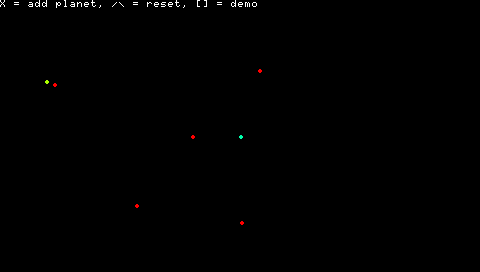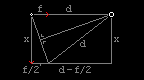# Blog - PSPlanets

Added on Monday, 2010-06-21 20:00 CEST in category Programming
PSPlanets is a crude simulator of Newtonian gravity. You can add planets and give them a mass, a position and a speed. And then gravity takes over :)You can add a planet by pressing X. The analog stick moves the planet around, and the L/R triggers change its mass. The lighter an object, the redder it becomes, the heavier, the bluer. Of course, heavier planets have more gravitational pull on other objects.

Once you've placed a planet by pressing X again, you can give it a specific direction and speed. If there are other planets already, pressing O will calculate the speed and direction for which the planet's orbit should be stable. This doesn't work well with moving planets, or when there's more than one heavy planet. However, the included demo, which can be run by pressing [] (square button), shows that an orbit around another planet that is itself in orbit is in fact possible.

Calculating a planet's stable orbit does work well with one heavy planet in the center. (The first planet to be placed is automatically centered.)

Technically the most interesting part was calculating stable orbits. The program's icon shows how a stable orbit can be calculated:There are two planets: the big, heavy planet on the right, and the newly placed, small planet on the left. The small planet has a downward momentum (x) and a gravitational force (f) acting on it. This force will change the planet's direction. The idea is in making the new direction perpendicular to the gravitational force of the big planet as seen from the small planet's new position, just like how it was before. This can be realized by optimizing x, using Pythagoras' theorem. See dev/stable.txt in the download for a more extensive explanation.

I know the formula may not be entirely mathematically sound, but for all practical purposes, it works just fine. There's one exception to this: if the force vector is bigger than the distance between the planets, the formula won't work anymore, because a perpendicular direction is impossible. This implies that the planet will be launched out of the planetary system.

Possible future enhancements could be:
• Calculate a stable orbit using all the other planets, not just the strongest one, for more accurate results;
• Also take all planets' speeds and directions into account, or even future positions.
• Zoom in and out using the L/R triggers;
• Alternate between clockwise and anti-clockwise stable orbits.

Also: this is my first proper application written in C++ :) The language has some pretty weird functions, like operator overloading, but so far I like it :)